# ECO 365 Economics Principles Editing and Proof Reading Services## Question 1

The demand curve demonstrates the relation between the price and the quantity of any product. Usually the demand and price have an inverse relationship wherein the quantity demanded for any project goes down with an increase in price. This is because when the price increases then the ability of people to consume a given good goes down and hence the overall demand for the product also goes down. This is the reason why the demand curve is always downward sloping. (Working, 1927)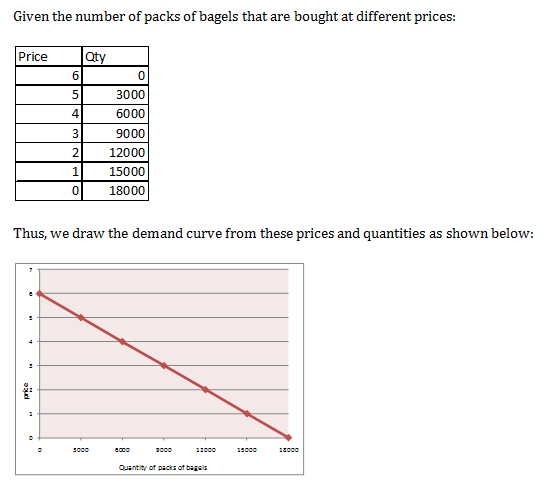The x axis represents the quantity of the packs of bagels and the y axis demonstrates the price at which the packs are purchased. It can be seen that the price and demand have a linear function but the relationship is inverse and hence the curve has a negative slope.

Usually the demand function is given by:

Qd = a + bP,

Where, Qd = quantity demanded

a = intercept value

b = slope

P = price of the item

Here, we will keep a and b as variables and hence, we would get the following equations:

0 = a + 6b………………..eqn 1

3000 = a +5b……………eqn 2

We thus try to solve these two equations to get the values of a and b,

a=-6b

i.e. 3000 = -6b + 5b

3000 = -b

b  = -3000, a = 18000

Therefore, the demand function can be written

Qd = 18000 – 3000P

The demand curve for the packs of bagels at different rates has been drawn. We now have to determine the point price elasticity of demand. Price elasticity of demand is nothing but a measure that helps in determining the sensitivity of the quantity that is demand as compared to the changes taking place in the price of the commodity. When the price elasticity is 1, then it means that the quantity demanded would change in equal proportions as per the demand and when the price elasticity is 0, it means that the quantity demanded would not change at all irrespective of the price changes. (Andrews, et. al 2010)

Price elasticity is usually denoted by (Fibich, et. al 2005)

PE = % change in quantity/ % change in price

= P/Q * (?Q/?P)

Demand function is given by:

Qd = 18000 -3000P

i.e. ?Q/?P = 0-3000 = -3000

At the point, where the price of bagels is \$3 per pack, the quantity is 9000

Hence, PE = 3/9000 * (-3000 )     = -1

At the price of \$3/pack, the demand is 9000 bagel packs

Hence, we calculate the revenue 9000*3 = \$27,000

When the price is increased to \$4/pack, the demand goes up to 6000 packs and the revenues are, 6000*4 = \$24,000

Hence, the total revenues go down by \$3000. As the price elasticity is negative, the total revenues would go down with an increase in price.

We again have to calculate the point price elasticity as shown in part b. We use the same approach as used in the b section of the question:

PE = % change in quantity/ % change in price

= P/Q * (?Q/?P)

Demand function is given by:

Qd = 18000 -3000P

i.e. ?Q/?P = 0-3000 = -3000

At the point, where the price of bagels is \$2 per pack, the quantity is 12000

Hence, PE = 2/12000 * -3000

= -0.5

When price is \$2 per pack, revenues = 2*12000 = \$24,000

When price is \$3 per pack, revenues = 3*9000 = \$27,000

The revenue would increase by \$3000, because the price elasticity is -0.5 because the price elasticity is relatively low and hence the revenues would go up because of increase in price. This correlation existing between the elasticity and the total revenues is something that has been proven practically by several studies and experiments. (Kara, et. al 2010)

Check more Economics Assignment Help>>>

### Question 2

The question gives equations for the weekly supply and demand of goods in thousands of units.

Weekly demand, P = 8 – Q

Weekly supply, P = 2 + Q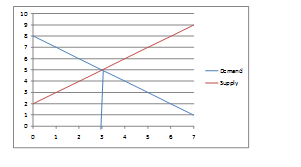We take these two equations and form a supply and demand curve as shown in the figure above. The x axis represents the quantity of the goods and the y axis demonstrates the price of the same. The demand curve is usually downward sloping because the quantity demanded goes down with an increase in price but the supply curve is usually upward sloping because the quantity supplied increases with an increase in price. (Dieffenbach, 1977)

The demand and supply curves can be given as shown in the diagram. The equilibrium is attained at the point where price is \$5 and the quantity is 3, the upper part of the blue region demonstrates the economic surplus that is generated at the market equilibrium.

Thus, we find the area of the triangle, i.e. ½* 3*3 = 4.5

Producer surplus = ½* 3*3 = 4.5

Total Economic surplus = 9

The government imposes an additional per unit tax to the sellers, which would lead to an increase in the price of the goods by \$2 per unit. The price increase would impact the supply curve because the suppliers would charge higher prices and hence the quantity supplied by them would increase but the quantity demanded would remain the same.

Tax per unit - \$2

Hence, new supply curve would be given as :

P= 2+ Q+ 2

P =4 + Q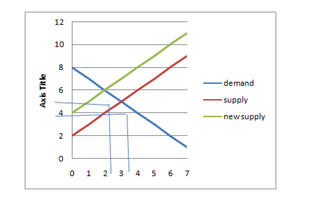New equilibrium, price = 6, quantity = 2

Consumer surplus = ½* 2*2= 2

Producer surplus = ½* 2*2 = 2

Total surplus = 4

Direct loss in surplus = 5

The tax leads to the generation of a particular amount of revenue because of increase in the selling price. Total revenue generated by tax = 2*2 = 4

Explore more onEconomics Assignment Help: Economic Liberalization>>>

### Question 3

Given the marginal, average variable and average total cost curves for a pizza seller: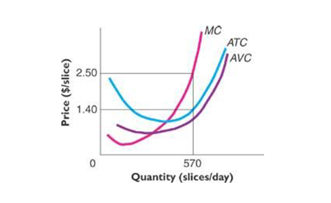The profit-maximizing level of output is at the point where the marginal cost equals the marginal revenue. This is the point where the demand curve would pass through both the marginal cost, as well as the marginal revenue curves. (Carbaugh, 2011) Here, the equilibrium is shown to be attained at 570 slices of pizza per day and hence that point the marginal revenues total the marginal costs.

Hence, profit-maximizing output = 570 slices/day

Average total costs at that point = \$1.40 /slice

Price of pizza = \$2.50 /slice

Hence, profit = (2.50- 1.40)= \$1.10 /slice= 1.10*570 = \$627.

This is the maximum profit that the pizza seller would obtain.

Given the marginal, average variable and average total cost curves for a pizza seller: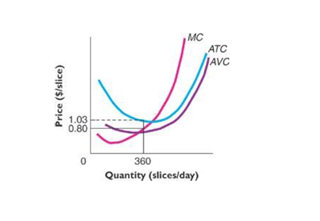The profit-maximizing level of output is at the point where the marginal cost equals the marginal revenue. This is the point where the demand curve would pass through both the marginal cost, as well as the marginal revenue curves. Here, the equilibrium is shown to be attained at 360 slices of pizza per day, where the marginal cost and the average variable costs curves coincide and hence that point the marginal revenues total the marginal costs.

Hence, profit-maximizing output = 360 slices/day

Average total costs at that point = \$1.03 /slice

Price of pizza = \$0.8 /slice

Hence, profit = (0.80- 1.03)= -\$0.23 /slice= -0.23*360 = -\$82.8

This is the maximum profit that the pizza seller would obtain, which means that the pizza seller is not making profit at all at the given costs. He is only making losses under the given conditions.

Get more help on Economic Impacts of Globalization>>>

### Question 4

Given gaming strategy:

A dating game between two players, A and B is given. The two strategies of the players are to either buy a ballet ticket or  to buy a football ticket. The matrix below demonstrates the payoffs in either of the situations for both the players. Each player is aware of the payoffs but does not know the strategy of the other person. Every player is rational and self-interested and thus choices made are completely unbiased.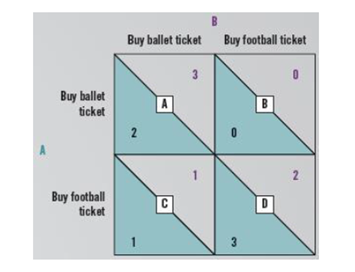No, none of the players have a dominant strategy in this case. A strategy is dominant when a player gets maximum payoff irrespective of the strategy chosen by the other player. But in this case, the payoff would ultimately depend on the strategy chosen by both the players put together. The strategy would be dominant only when both the players select the same strategy. (Mizukami, et. al 2007) And hence in the given strategy, none of the players have a dominant strategy.

There are two possible equilibriums in the given gaming strategy. The first equilibrium arises when both the players buy ballet ticket and the second one arises when both the players buy football ticket. In such situations, the payoff for both the players becomes maximum and hence these are known as the equilibrium strategies. (Antal, et. al 2009) In these strategies, neither of the players would benefit by changing its strategy. While adopting all other strategies, the players can enhance their pay offs by selecting some other strategy.

No the game is not a prisoner’s dilemma because in prisoner’s dilemma, both the players have a dominant strategy that leads to maximum payoffs for both of them but such a condition is not prevalent in this strategy and hence it is not a prisoner’s dilemma. Prisoner’s dilemma is a very commonly used and tested gaming strategy and represents the way players can have dominant strategies and can use them for maximum payoffs. (Roth, et. al 1978)

### Conclusion

By this exercise, we have analyzed the different relations between the supply and demand of different goods, their prices and quantities. Examples are taken to numerically illustrate the functioning of the demand and supply curves and how the changes in different factors like price, tax etc. can lead to overall economic changes. A gaming strategy is also taken as an example to illustrate how the strategies work and what can get the same in equilibrium.

### References

• Working, E.J. (1927), What do Statistical “Demand Curves” Show?, The Quarterly Journal of Economics,vol. 41, issue 2, pp. 212-235
• Andrews, T., & Benzing (2010), Simplifying the Price Elasticity of Demand, Journal for Economic Educators,vol.10(1)
• Fibich, G., Gavious, A., & Lowengart, O. (2005), Journal of the Academy of Marketing Science,vol. 33, no.1, pp. 66-78
• Dieffenbach, B.C. (1977), Aggregate Effective Demand and Supply Curves, Economic Inquiry,vol. 15, issue 2, pp.199-212
• Carbaugh, R., & Prante, T. (2011), A Primer on Profit Maximization, Journal for Economic Educators,vol. 11(2)
• Mizukami, H., & Wakayama, T. (2007), Dominant Strategy Implementation in Economic Environments, ISER Discussion Paper, Institute of Social and Economic Research, Osaka University
• Antal, T., Traulsen, A., Ohtsuki, H., Tarnita, C.E., & Nowak, M.A. (2009), Mutation-selection equilibrium in games with multiple strategies, Journal of Theoretical Biology,vol. 258, pp. 614-622
• Roth, A.E., & Murnighan, J.K. (1978), Equilibrium Behavior and Repeated Play of the Prisoner’s Dilemma, Journal of Mathematical Psychology,vol. 17, pp. 189-198